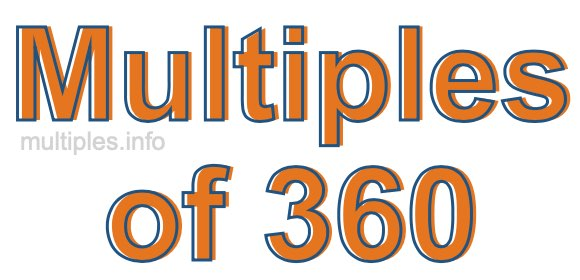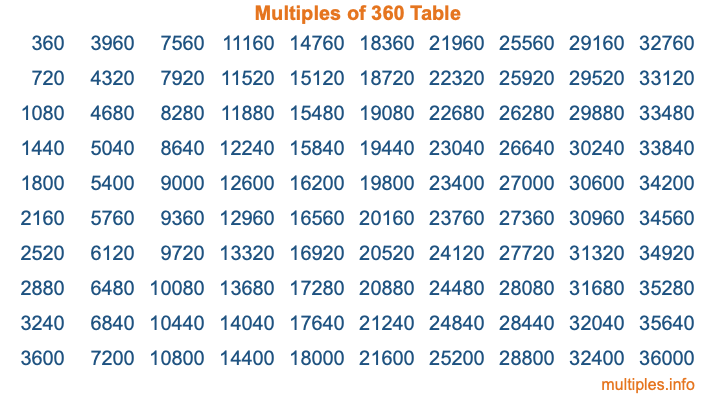Multiples of 360Welcome to the Multiples of 360 page. Here we will first teach you everything you will ever need to know about the multiples of 360, and then give you a study guide summary of everything we taught you to make sure you remember it all. Use this page to look up facts and learn information about the multiples of 360. This page will make you a multiples of three hundred sixty expert!

Definition of Multiples of 360
Multiples of 360 are all the numbers that when divided by 360 equal an integer. Each of the multiples of 360 are called a multiple. A multiple of 360 is created by multiplying 360 by an integer.

Therefore, to create a list of multiples of 360, you start with 1 multiplied by 360, then 2 multiplied by 360, then 3 multiplied by 360, and so on for as long as you want. Thus, the list of the first five multiples of 360 is 360, 720, 1080, 1440, and 1800. To see a larger list of multiples of 360, see the printable image of Multiples of 360 further down on this page. We also have a category where you can choose any nth multiple of 360.

Multiples of 360 Checker
The Multiples of 360 Checker below checks to see if any number of your choice is a multiple of 360. In other words, it checks to see if there is any number (integer) that when multiplied by 360 will equal your number. To do that, we divide your number by 360. If the the quotient is an integer, then your number is a multiple of 360.

Is  a multiple of 360?

Least Common Multiple of 360 and ...
A Least Common Multiple (LCM) is the lowest multiple that two or more numbers have in common. This is also called the smallest common multiple or lowest common multiple and is useful to know when you are adding our subtracting fractions. Enter one or more numbers below (360 is already entered) to find the LCM.

Check out our LCM Calculator if you need more details about the Least Common Multiple or if you need the LCM for different numbers for adding and subtraction fractions.

nth Multiple of 360
As we stated above, 360 is the first multiple of 360, 720 is the second multiple of 360, 1080 is the third multiple of 360, and so on. Enter a number below to find the nth multiple of 360.

th multiple of 360

Multiples of 360 vs Factors of 360
360 is a multiple of 360 and a factor of 360, but that is where the similarities end. All postive multiples of 360 are 360 or greater than 360. All positive factors of 360 are 360 or less than 360.

Below is the beginning list of multiples of 360 and the factors of 360 so you can compare:

Multiples of 360: 360, 720, 1080, 1440, 1800, etc.

Factors of 360: 1, 2, 3, 4, 5, 6, 8, 9, 10, 12, 15, 18, 20, 24, 30, 36, 40, 45, 60, 72, 90, 120, 180, 360

As you can see, the multiples of 360 are all the numbers that you can divide by 360 to get a whole number. The factors of 360, on the other hand, are all the whole numbers that you can multiply by another whole number to get 360.

It's also interesting to note that if a number (x) is a factor of 360, then 360 will also be a multiple of that number (x).

Multiples of 360 vs Divisors of 360
The divisors of 360 are all the integers that 360 can be divided by evenly. Below is a list of the divisors of 360.

Divisors of 360: 1, 2, 3, 4, 5, 6, 8, 9, 10, 12, 15, 18, 20, 24, 30, 36, 40, 45, 60, 72, 90, 120, 180, 360

The interesting thing to note here is that if you take any multiple of 360 and divide it by a divisor of 360, you will see that the quotient is an integer.

Multiples of 360 Table
Below is an image of the first 100 multiples of 360 in a table. The table is in chronological order, column by column. The first column has the first ten multiples of 360, the second column has the next ten multiples of 360, and so on.The Multiples of 360 Table is also referred to as the 360 Times Table or Times Table of 360. You are welcome to print out our table for your studies.

Negative Multiples of 360
Although not often discussed or needed in math, it is worth mentioning that you can make a list of negative multiples of 360 by multiplying 360 by -1, then by -2, then by -3, and so on, to get the following list of negative multiples of 360:

-360, -720, -1080, -1440, -1800, etc.

Multiples of 360 Summary
Below is a summary of important Multiples of 360 facts that we have discussed on this page. To retain the knowledge on this page, we recommend that you read through the summary and explain to yourself or a study partner why they hold true.

There are an infinite number of multiples of 360.

A multiple of 360 divided by 360 will equal a whole number.

360 divided by a factor of 360 equals a divisor of 360.

The nth multiple of 360 is n times 360.

The largest factor of 360 is equal to the first positive multiple of 360.

360 is a multiple of every factor of 360.

360 is a multiple of 360.

A multiple of 360 divided by a divisor of 360 equals an integer.

360 divided by a divisor of 360 equals a factor of 360.

Any integer times 360 will equal a multiple of 360.

Multiples of a Number
Here you can get the multiples of another number, all with the same attention to detail as we did for multiples of 360 on this page.

Multiples of
Multiples of 361
Did you find our page about multiples of three hundred sixty educational? Do you want more knowledge? Check out the multiples of the next number on our list!